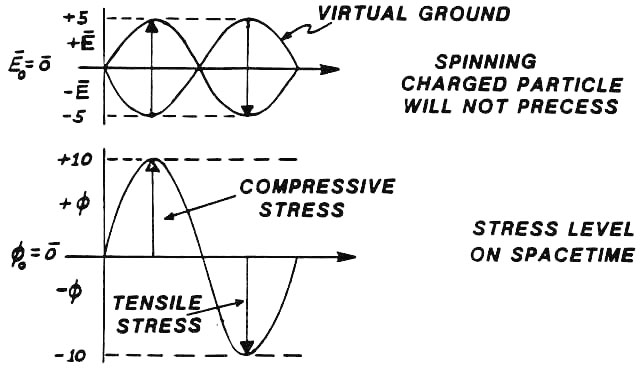SCALAR Ø-WAVE PRODUCTION

SLIDE 23We now visualize the formation of waves of pure stress in the spacetime medium (in the vacuum). These we call scalar EM waves, Tesla waves, electrogravitational waves, longitudinal EM waves, waves of pure potential, electrostatic/magnetostatic waves, and zero-vector EM waves. All these terms are synonymous. Each sheds its own particular light upon the nature of these waves or of their original discoverer, Nikola Tesla. We use a gedankenexperiment, or thought experiment, in which we can be "perfect." Thus we have two single-frequency EM sine waves whose E-field components are shown on the slide. The two waves are of the same frequency, traveling together in the same direction, and superposed 180 degrees out of phase with each other. In this case, at any spatial point, the summation E and B fields are vector zeros. However, if we plot the spatiotemporal (vacuum) stress induced by the wave, we see that it constitutes a sine wave, with compressive stress in one half cycle and tensile stress in the second half cycle. Rigorously this defines a "longitudinal" EM wave -- or what Tesla called a "sound wave in the nonmaterial ether.’’ Also, rigorously it is a gravitational wave, for it is a wave of the curvature of spacetime (nonlinearity of vacuum) itself. That is, what is changing in the wave is the vacuum stress, or intensity of the virtual particle flux of vacuum. That is identical to changing the curvature of spacetime. In one half-cycle, spacetime is curved positively. In the second half-cycle, spacetime is curved negatively. Since the increase or decrease of the intensity of virtual particle flux (vis-a-vis the ambient vacuum’s virtual flux intensity) represents electrical charge, then in one half-cycle negative charge is represented, and in the other half-cycle positive charge is represented. This directly explains the "positron-electron pair" representation of an electromagnetic photon -- the "photon" being one wavelength. In one half-cycle, time moves slower. In the second half-cycle, time moves faster. Thus scalar waves can also be considered to be "tempic" waves (to use Wilbur Smith’s term), or oscillations of the rate of flow of time itself, about the ambient rate of time flow. Since these oscillations are variations in the curvature of spacetime, then they represent gravitational or force-generating waves, when coupled to a mass. Variations in the rate of flow of time produce force, just as any other type of curvature in space time does. Previous Slide